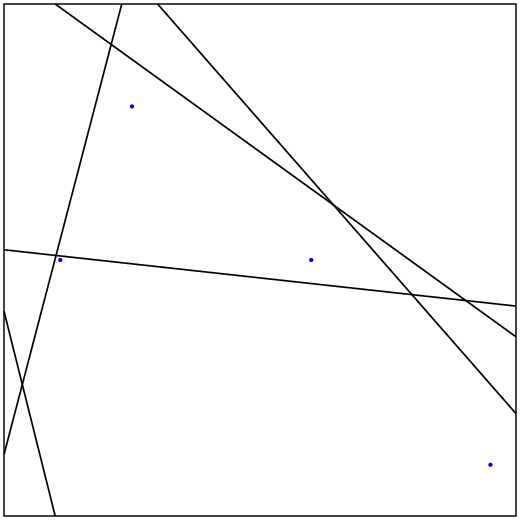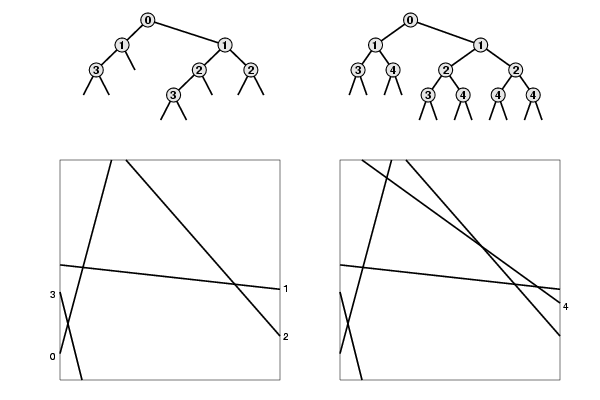## Point Location

Write a program to solve the point location problem among line arrangements in the plane. Your program should build a data structure from a set of lines that allows it to, when given a pair of points, quickly determine whether any of the lines goes between the two points. The brute-force solution to this problem is to test that both points are on the same side of each of the N lines. The goal of this assignment is to build a data structure that cuts the number of lines to be tested down to be proportional to log N.

Input format. For simplicity, you may assume that all the lines cross the unit square (where both coordinates are between 0 and 1), and that you only have to work with points and line segments in that region. Use the following input format:

 ```5 0.00 0.12 0.23 1.00 1.00 0.41 0.00 0.52 1.00 0.20 0.30 1.00 0.00 0.40 0.10 0.00 1.00 0.35 0.10 1.00 0.25 0.80 0.60 0.50 0.95 0.10 0.11 0.50 ```The input begins with an integer N, then N lines, each defined by four real numbers that give the endpoints of the line segments. Since they represent intersections with the edge of the unit square, each line segment endpoint has at least one coordinate with value 0 or 1. Following the N lines is a sequence of pairs of points (also four reals per input line).

You do not have to worry about handling all possible degenerate cases, but you shouldn't completely ignore them, either. Certainly you will want to get your program working on straightforward inputs before worrying about degenerate examples such as three lines intersecting in a point, or where precision in the calculation affects a decision. In your design, you should be cognizant of places where your program might be exposed to trouble for a degenerate case, whether or not you get around to fixing it up. In your readme.txt file, be sure to explain which kinds of input you have thought about.

A possible solution method. An arrangement is the planar subdivision defined by the lines. The diagram below is the arrangement for the set of lines given above. Every point in the unit square falls into some region; every region is bounded by some subset of the line segments. Your task is to be able to determine quickly whether or not two given input points fall into the same region or not.One way to solve this problem is to build a binary tree with internal nodes corresponding to line segments and external nodes corresponding to regions in the plane, as shown in the example. This is similar to a 2D tree. A search in this tree involves comparing a point against the line segment at the root, then going left if it is on one side and right if it is on the other side. If the search for two points ends up at the same external node, they must be in the same region. There are at most N*N regions and one external node corresponding to each region, so we expect that the time should be proportional to log(N*N) = 2 log N for random data. Figuring out how to build this tree for arbitrary lines is the main challenge of this assignment.

Getting started. You will need some geometry primitives: a test whether a point is on one side of a line or the other and a routine to compute the intersection point of two lines. The former is essentially the ccw() function from lecture and the book. The latter requires some high-school geometry, coupled with some computer-science considerations having to do with precision and degenerate cases (lines consisting of a single point, parallel lines, and so forth).

Output. For each pair of query points, indicate whether or not they are separated by a line, and if they are, print out one such line. Also, instrument your program to count the number of external nodes and the average path length (external path length divided by number of external nodes) for the trees that you construct. Include a table with these numbers, and a discussion of how they grow, in your writeup.

Deliverables. Organize your program into natural modules, e.g., Point.java, LineSegment.java, and PointLocator.java. We will assume that your main client (that reads in the lines and processes the point pairs) is in PointLocator.java.